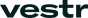# Understanding Delta in Derivatives Trading: A Comprehensive Guide

Delta is a crucial concept in the world of derivatives trading, particularly in options trading. It plays a significant role in determining the price movement of an option concerning changes in the underlying asset's price. As a trader or investor, having a thorough understanding of delta and its implications can significantly enhance your trading strategy and decision-making. In this article, we'll delve into what delta is, how it's calculated, and its relevance in derivatives trading.

## What is Delta?

Delta, represented by the Greek letter Δ, is a measurement used in finance to describe the rate of change of an option's price in relation to the price movement of the underlying asset. In simple terms, delta indicates how much the option price is likely to change for a one-point movement in the underlying asset's price.

Delta values range from 0 to 1 for call options and from -1 to 0 for put options. A delta of 0.5 (or -0.5 for puts) implies that for every one-point increase (or decrease) in the underlying asset's price, the option price will increase (or decrease) by 0.5.

## Calculating Delta

The formula to calculate delta for a call option is:

Call Delta=∂Call Price∂Underlying Asset PriceCall Delta=∂Underlying Asset Price∂Call Price​

And for a put option:

Put Delta=∂Put Price∂Underlying Asset PricePut Delta=∂Underlying Asset Price∂Put Price​

The delta of a call option typically ranges from 0 to 1, while for a put option, it ranges from -1 to 0. A delta of 0.5 indicates that the option price will increase by 0.5 for every one-point increase in the underlying asset's price.

## Understanding Delta's Significance

Delta has several implications for options traders:

1. Price Sensitivity: Delta helps traders understand how much the option price is affected by changes in the underlying asset's price. Options with higher deltas are more sensitive to price changes, and their prices move in tandem with the underlying asset's price.
2. Hedging: Delta is vital for creating delta-neutral strategies. Traders often offset the delta of an option by taking an opposite position in the underlying asset. This allows them to hedge against price movements.
3. Probability Estimation: Delta also provides an estimation of the probability that an option will expire in-the-money. A delta of 0.7, for instance, suggests a 70% chance the option will be in-the-money at expiration.
4. Leverage Management: Understanding delta helps in managing leverage. Options with higher delta values offer more leverage but also entail higher risk.

## Factors Affecting Delta

Delta is influenced by several factors:

• Moneyness: Delta is highest for at-the-money options and decreases as the option moves in-the-money or out-of-the-money.
• Time to Expiration: Delta tends to increase as expiration approaches, particularly for at-the-money options.
• Volatility: Higher volatility generally increases the delta of both call and put options.

## Conclusion

Delta is a vital tool for options traders, helping them understand the sensitivity of option prices to changes in the underlying asset's price. By grasping delta's implications and its calculation, traders can make informed decisions, manage risks, and construct effective trading strategies. It's crucial to continuously monitor delta and its influencing factors to navigate the dynamic landscape of derivatives trading effectively.

# ‍